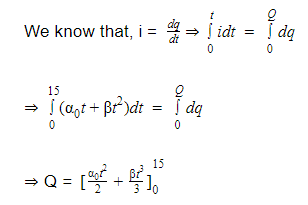# A current through a wire depends on time as i = α0t + βt2 where α0 = 20 A/s and β = 8 As-2. Find the charge crossed through a section of the wire in 15 s.

a. 2100 C

b. 260 C

c. 2250 C

d. 11250 C

Given: i = α0t + βt2

α = 20 A/s and β = 8As-2

t = 15 sec⇒ Q = 20×15×15 / 2 + 8×15×15×15 / 3 – 0

⇒ Q = 11250 C(0)(0)